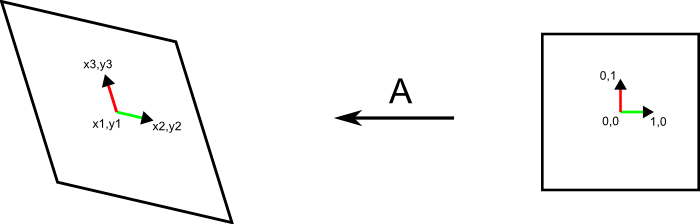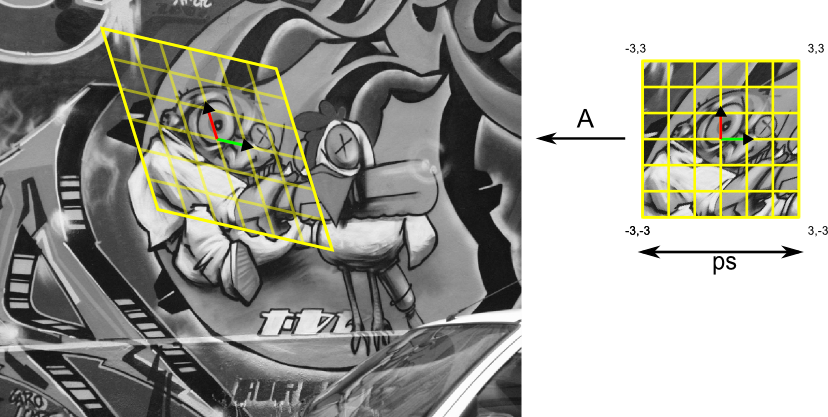Warning

# Introduction to MATLAB

MATLAB will be used for the MPV labs. In case you are not familiar with matlab, study the following parts of the "Getting started with MATLAB (MathWorks)":

• Matrices and Arrays - (Expressions, Working with Matrices, More About Matrices and Arrays)
• Graphics - (Editing Plots, Mesh and Surface Plots, Images)
• Programming - (Flow Control, Other Data Structures, Scripts and Functions)

(home study)

Other useful tutorials are:

# Basics of Image Processing in Matlab

## Convolution, Image Smoothing and Gradient

• The Gaussian function is often used in image processing as a low pass filter for noise reduction, or as a windowing function weighting points in a neighbourhood. Implement the function G=gauss(x,sigma) that computes values of a (1D) Gaussian with zero mean and variance $\sigma^2$:
$$G = \frac{1}{\sqrt{2\pi}\sigma}\cdot e^{-\frac{x^2}{2\sigma^2}}$$
in points specified by vector x.
• Implement function D=dgauss(x,sigma) that returns the first derivative of a Gaussian
$$\frac{d}{dx}G(x) = \frac{d}{dx}\frac{1}{\sqrt{2\pi}\sigma}\cdot e^{-\frac{x^2}{2\sigma^2}} = -\frac{1}{\sqrt{2\pi}\sigma^3}\cdot x\cdot e^{-\frac{x^2}{2\sigma^2}} = -\frac{x}{\sigma^2}G(x)$$
in points specified by vector x.
• Get acquainted with the function conv2.
• The effect of filtering with Gaussian and its derivative can be best visualized using an impulse (1-nonzero-pixel) image:
sigma = 6.0;
x = [-ceil(3.0*sigma):ceil(3.0*sigma)];
G = gauss(x, sigma);
D = dgauss(x, sigma);
imp = zeros(49); imp(25,25) = 1.0;
out = conv2(D, G, imp, 'same');
...

imagesc(out); % or surf(out);
try to find out impulse responses of other combinations of the Gaussian and its derivatives.
• Write a function out=gaussfilter(in,sigma) that implements smoothing of an input image in with a Gaussian filter of width 2*ceil(sigma*3.0)+1 and variance $\sigma^2$ and returns the smoothed image out (e.g. Lenna). Exploit the separability property of Gaussian filter and implement the smoothing as two convolutions with one dimensional Gaussian filter (see function conv2).
• Modify function gaussfilter to a new function [dx,dy]=gaussderiv(in,sigma) that returns the estimate of the gradient (gx, gy) in each point of the input image in (MxN matrix) after smoothing with Gaussian with variance $\sigma^2$. Use either first derivative of Gaussian or the convolution and symmetric difference to estimate the gradient.
• Implement function [dxx,dxy,dyy]=gaussderiv2(in,sigma) that returns all second derivatives of input image in (MxN matrix) after smoothing with Gaussian of variance $\sigma^2$.

## Geometric Transformations and Interpolation of the Image

• Implement function A=affine(x1,y1,x2,y2,x3,y3) that returns 3×3 transformation matrix A which transforms point in homogeneous coordinates from canonical coordinate system into image: (0,0,1)→(x1,y1,1), (1,0,1)→(x2,y2,1), (0,1,1)→(x3,y3,1).• Write function out=affinetr(in,A,ps,ext) that warps a patch from image in (MxN matrix) into canonical coordinate system. Affine transformation matrix A (3×3 elements) is a transformation matrix from the canonical coordinate system into image from previous task. The parameter ps defines the dimensions of the output image (the length of each side) and ext is a real number that defines the extent of the patch in coordinates of the canonical coordinate system. E.g. out=affinetr(in,A,41,3.0), returns the patch of size 41×41 pixels that corresponds to the rectangle (-3.0,-3.0)x(3.0,3.0) in the canonical coordinate system. Top left corner of the image has coordinates (0,0). Use bilinear interpolation for image warping. Check the functionality on image.Upload all the Matlab functions implemented in this lab: gauss.m, dgauss.m, gaussfilter.m, gaussderiv.m, gaussderiv2.m, affine.m and affinetr.m into the upload system in a .zip archive. Keep all functions in the root of the .zip archive together with all helper functions required. Follow closely the specification and order of the input/output arguments.